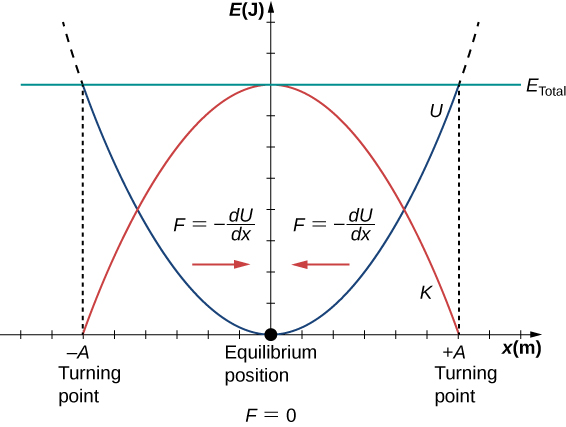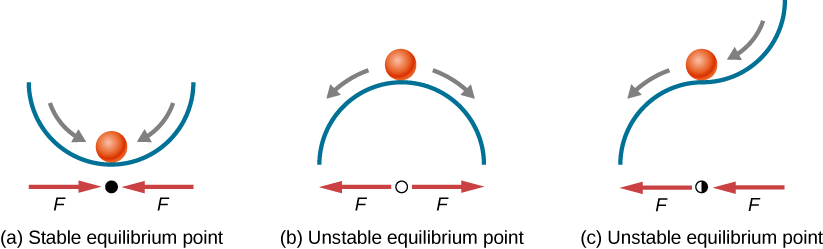# 15.2 Energy in simple harmonic motion  (Page 3/8)

 Page 3 / 8

## Oscillations about an equilibrium position

We have just considered the energy of SHM as a function of time. Another interesting view of the simple harmonic oscillator is to consider the energy as a function of position. [link] shows a graph of the energy versus position of a system undergoing SHM.A graph of the kinetic energy (red), potential energy (blue), and total energy (green) of a simple harmonic oscillator. The force is equal to F = − d U d x . The equilibrium position is shown as a black dot and is the point where the force is equal to zero. The force is positive when x < 0 , negative when x > 0 , and equal to zero when x = 0 .

The potential energy curve in [link] resembles a bowl. When a marble is placed in a bowl, it settles to the equilibrium position at the lowest point of the bowl $\left(x=0\right)$ . This happens because a restoring force    points toward the equilibrium point. This equilibrium point is sometimes referred to as a fixed point . When the marble is disturbed to a different position $\left(x=+A\right)$ , the marble oscillates around the equilibrium position. Looking back at the graph of potential energy, the force can be found by looking at the slope of the potential energy graph $\left(F=-\frac{dU}{dx}\right)$ . Since the force on either side of the fixed point points back toward the equilibrium point, the equilibrium point is called a stable equilibrium point    . The points $x=A$ and $x=\text{−}A$ are called the turning points . (See Potential Energy and Conservation of Energy .)

Stability is an important concept. If an equilibrium point is stable, a slight disturbance of an object that is initially at the stable equilibrium point will cause the object to oscillate around that point. The stable equilibrium point occurs because the force on either side is directed toward it. For an unstable equilibrium point, if the object is disturbed slightly, it does not return to the equilibrium point.

Consider the marble in the bowl example. If the bowl is right-side up, the marble, if disturbed slightly, will oscillate around the stable equilibrium point. If the bowl is turned upside down, the marble can be balanced on the top, at the equilibrium point where the net force is zero. However, if the marble is disturbed slightly, it will not return to the equilibrium point, but will instead roll off the bowl. The reason is that the force on either side of the equilibrium point is directed away from that point. This point is an unstable equilibrium point.

[link] shows three conditions. The first is a stable equilibrium point (a), the second is an unstable equilibrium point (b), and the last is also an unstable equilibrium point (c), because the force on only one side points toward the equilibrium point.Examples of equilibrium points. (a) Stable equilibrium point; (b) unstable equilibrium point; (c) unstable equilibrium point (sometimes referred to as a half-stable equilibrium point).

The process of determining whether an equilibrium point is stable or unstable can be formalized. Consider the potential energy curves shown in [link] . The force can be found by analyzing the slope of the graph. The force is $F=-\frac{dU}{dx}.$ In (a), the fixed point is at $x=0.00\phantom{\rule{0.2em}{0ex}}\text{m}\text{.}$ When $x<0.00\phantom{\rule{0.2em}{0ex}}\text{m,}$ the force is positive. When $x>0.00\phantom{\rule{0.2em}{0ex}}\text{m,}$ the force is negative. This is a stable point. In (b), the fixed point is at $x=0.00\phantom{\rule{0.2em}{0ex}}\text{m}\text{.}$ When $x<0.00\phantom{\rule{0.2em}{0ex}}\text{m,}$ the force is negative. When $x>0.00\phantom{\rule{0.2em}{0ex}}\text{m,}$ the force is also negative. This is an unstable point.

a length of copper wire was measured to be 50m with an uncertainty of 1cm, the thickness of the wire was measured to be 1mm with an uncertainty of 0.01mm, using a micrometer screw gauge, calculate the of copper wire used
If centripetal force is directed towards the center,why do you feel that you're thrown away from the center as a car goes around a curve? Explain
Which kind of wave does wind form
calculate the distance you will travel if you mantain an average speed of 10N m/s for 40 second
hw to calculate the momentum of the 2000.0 elephant change hunter at a speed of 7.50 m/s
how many cm makes 1 inches
2.5
omwoyo
2.54cm=1inche
omwoyo
how do we convert from m/s to km/hr
When paddling a canoe upstream, it is wisest to travel as near to the shore as possible. When canoeing downstream, it may be best to stay near the middle. Explain why?
Explain why polarization does not occur in sound
one ship sailing east with a speed of 7.5m/s passes a certain point at 8am and a second ship sailing north at the same speed passed the same point at 9.30am at what distance are they closet together and what is the distance between them then
density of a subtance is given as 360g/cm,put it in it s.i unit form
if m2 is twice of m1. find the ration of kinetic energy in COM system to lab system of elastic collision
What is a volt equal to?
list and explain the 3 ways of charging a conductor
conduction convention rubbing
Asdesaw
formula of magnetic field
why polarization does not occur in sound
Integral of a vector
define surface integral of a vector?
Rahat

#### Get Jobilize Job Search Mobile App in your pocket Now!By RhodesByBy Stephen VoronBy Stephen VoronBy OpenStaxBy Stephen VoronBy Naveen TomarBy Monty HartfieldBy OpenStaxBy Stephen Voron